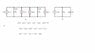# Equivalent Circuits: How Do (a) & (b) Compare?

• Miike012

#### Miike012

How are the circuits (a) and (b) equivalent?
i0 is the sum of two currents that flow from high to low potential (flow of positive charge)
So why does i0 not flow from high to low pot?

#### Attachments

•CCQ.jpg
10.9 KB · Views: 389
Last edited:
Note that this circuit is parallel, and hence we know that the voltage is the same all along the upper bus (or node if you prefer), and along the lower bus. They have specified that the upper node is +. Thus all of the interesting action is in the current distributions. Since the current sources have fixed currents ... we are most interested in the currents flowing through the two resistors.

The first equation is KCL with all of the currents originating at the upper node.

The second equation is a rearrangement, putting the currents that pass through the resistors on one side, and the current sources on the other.

The third equation is a definition which combines the current sources.

The drawing is a simplified circuit - all of the current sources have been combined - and the voltage difference between the nodes has been noted in a different place. Currents i2 and i5 have not been labeled, but they belong with the two resistors.

Yes: "i0 is the sum of two currents" ... and you can see that the arrows for i2 and i5 should go from top to bottom "from high to low potential (flow of positive charge)".

"So why does i0 not flow from high to low pot?" ... the current generated at the + terminal must all return to the - terminal if there is no charge storage: it flows from the generator to the + node, then down through the two resistors (you need to put the current flow arrows on the diagram), to the lower node, and then returns to the - terminal of the current generator. "So why does i0 not flow from high to low pot?" - it does - and then returns to the generator.

The voltage across the resistors is determined by v(t) because an ideal voltage source will impose it's voltage on anything.

If you have something that controls the voltage across a resistor the current through it will also be controlled. See ohms law.

If the voltage and current through the resistors is controlled by v(t) then I3(t) and I4(t) and i6(t) have no effect on the voltage or current through the resistors.

If you are only interested in the voltage and current through the resistors you can therefore model the circuit as either a single voltage source or as a single current source that performs the same function as V(t). They have chosen a current source io(t).

Lets say i4(t) was a massive current source of 1,000,0000 amps. That still wouldn't effect the current through the resistors because v(t) controls the voltage across them. So where does all the current go? The answer is that most of it will go through the voltage source v(t). An ideal voltage source _will_ source or sink whatever current is necessary to maintain it's output voltage.

Last edited: